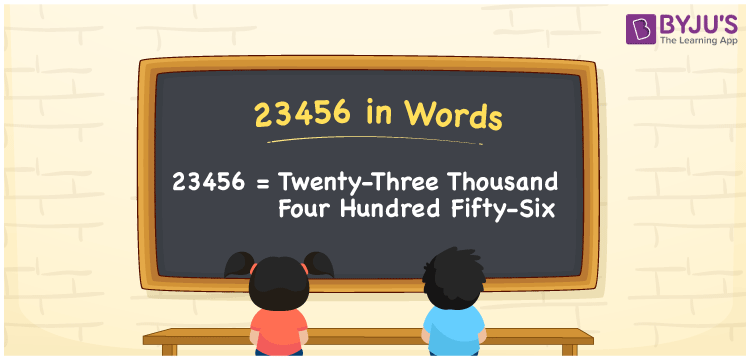# 23456 in Words

23456 in words is Twenty-three thousand four hundred fifty-six. We can convert the number 23456 into words using a place value chart. If you bought a new cupboard that costs Rs. 23456, then you can say, “I bought a new cupboard worth Twenty-three thousand four hundred fifty-six rupees”. Also, 23456 is a cardinal number since it denotes a certain measure or quantity.

 23456 in words Twenty-three thousand four hundred fifty-six Twenty-three thousand four hundred fifty-six in Numbers 23456

## 23456 in English words

Generally, we write numbers in words using the English alphabet. Thus, we can read 23456 in English as “Twenty-three thousand four hundred fifty-six”.## How to Write 23456 in Words?

As we know, 23456 is a five-digit number so, we need to make a five-column place value chart to write the number 23456 in words. This can be shown as follows.

 Ten thousand Thousands Hundreds Tens Ones 2 3 4 5 6

Here, ones = 6, tens = 5, hundred = 4, thousands = 3, ten thousands = 2

By expanding the above digits according to their place values, we get;

2 × Ten thousand + 3 × Thousand + 4 × Hundred + 5 × Ten + 6 × One

= 2 × 10000 + 3 × 1000 + 4 × 100 + 5 × 10 + 6 × 1

= 20000 + 3000 + 400 + 50 + 6

= Twenty thousand + Three thousand + Four hundred + Fifty + Six

= Twenty-three thousand four hundred fifty-six

Hence, 23456 in words = Twenty-three thousand four hundred fifty-six.

As we know, 23456 is a natural number that is the successor of 23455 and the predecessor of 23457.

23456 in words – Twenty-three thousand four hundred fifty-six

Is 23456 an odd number? – No

Is 23456 an even number? – Yes

Is 23456 a perfect square number? – No

Is 23456 a perfect cube number? – No

Is 23456 a prime number? – No

Is 23456 a composite number? – Yes

## Frequently Asked Questions on 23456 in Words

Q1

### How do you say 23456 in words?

We can spell the number 23456 in words as “Twenty-three thousand four hundred fifty-six”.
Q2

### How to write Rs. 23456 in words on a cheque?

On a cheque, we can mention the amount Rs. 23456 in words as “Twenty-three thousand four hundred fifty-six rupees only”.
Q3

### Find the value of Twenty-three thousand four hundred fifty-six minus Twenty thousand.

Twenty-three thousand four hundred fifty-six minus Twenty thousand = 23456 – 20000 = 3456 = Three thousand four hundred six.